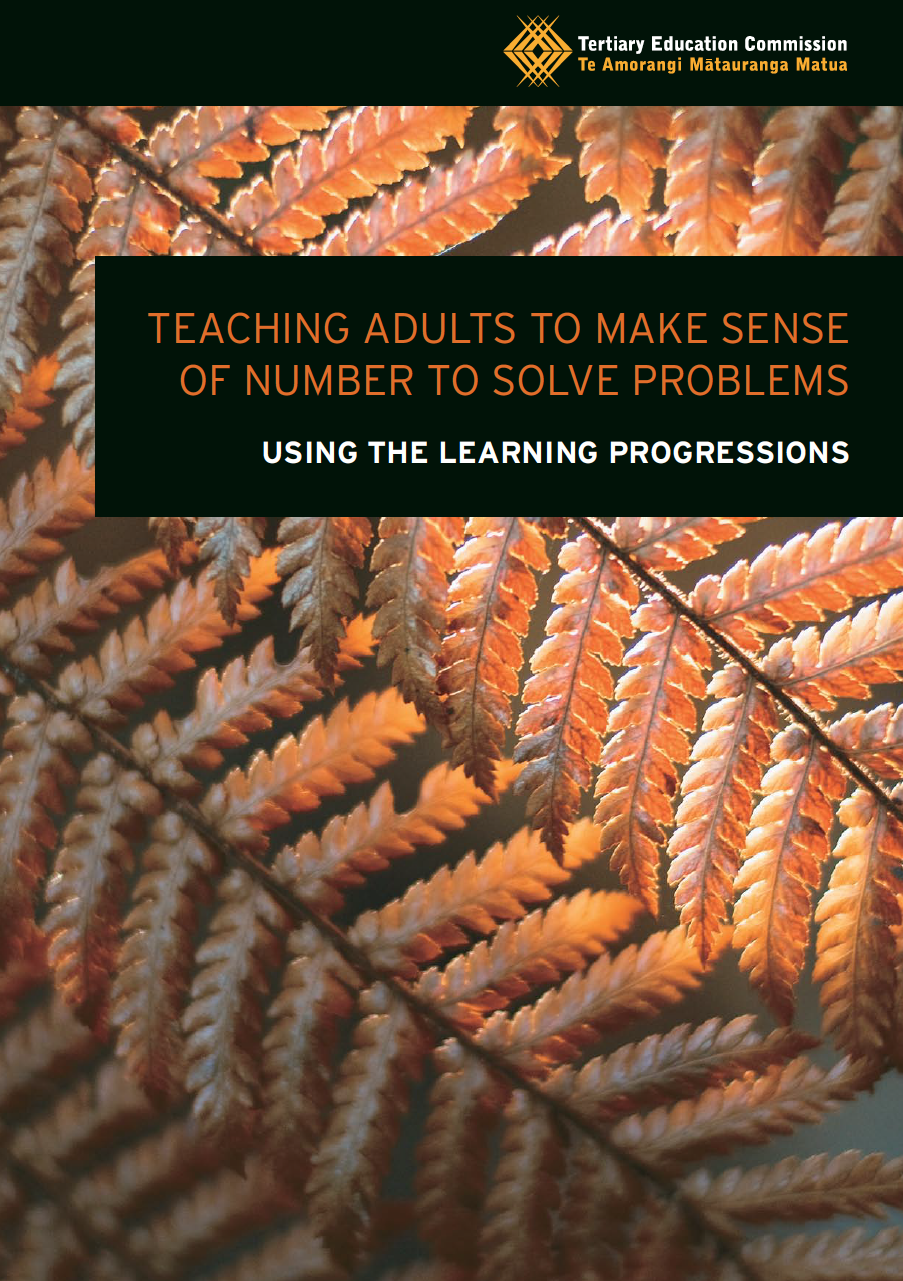# TEACH: Cheat sheet for number *knowledge* activities from the Learning ProgressionsThis is part two of two parts. Here we look at numeracy activities that relate to Number knowledge. This includes:

• Number sequence knowledge
• Place value knowledge
• Number facts knowledge

You can find numeracy activities that relate to Number Strategies on a different page.

You may already have downloaded this resource shown in the image above. If you haven’t and you want to click the link below:

These resources are filled with activities and ideas that you can use or adapt. But navigating them can be tricky. So we designed a cheat sheet.

# Cheat Sheet for Number Knowledge Activities

Here’s how to use it. Just look down the list at the second column. This tells you in one sentence what learners actually have to do in the activity.

From there, you can look at the name of the activity, find the page number in the resource, and see which progressions it lines up with.

Things to remember:

• Some activities cut across multiple progressions. This is fine. Just make sure you know what your focus is. Refer back to your learning outcomes.
• These numeracy activities are specific to certain steps. Make sure that you know what step your learners are at first.
• Not all the activities will be useful to you and your situation. If you do decide to take the time to look through these, you might want to rate them for yourself. In other words, can you use this?
• You don’t have to use these. If you have your own ideas then go with that. But remember, if you need inspiration or want to look at activities that were written by experts then look here.

You can download the cheat sheets for all three strategy progressions as a single PDF below or just read on.

## Number Sequence Knowledge Activity Cheat Sheet

#### Can I use it? Rate: ✓ ? ✗

Numbers to 100 Read and write numbers from 0 to 100; position the numbers on an empty number line. Step 2 P. 67
Understanding fractions I Order fractions with the same bottom number (denominator) by cutting, naming and ordering strips of paper. Step 3 P. 69
Understanding fractions II Order unit fractions (where the bottom number is different); understand that as the bottom number gets larger, the size of the fraction gets smaller. Step 4 P. 70
Understanding fractions III

Use fraction in division problems where the numbers do not divide evenly. Step 4 P. 71
Decimal number place value Develop their understanding of the place value system to include decimal numbers including tenths, hundredths and thousandths; name any decimal number in tenths, hundredths and thousandths. Step 5 P. 78

## Place Value Knowledge Activity Cheat Sheet

#### Can I use it? Rate: ✓ ? ✗

Introducing place value Work with a place value chart to develop their understanding that our number system is based on the number 10; Understand that the place of a digit in a number indicate its value. Step 2- 3 P. 73
Whole number place value Extend their understanding of place value by adding and subtracting 1, 10, 100 and 1000 from a given four-digit number. Step 3 P. 76
Decimal number place value Develop their understanding of the place value system to include decimal numbers including tenths, hundredths and thousandths; name any decimal number in tenths, hundredths and thousandths. Step 5 P. 78
Connecting percentages, decimals
and fractions
Explore connections between percentages, decimals and fractions; develop mental strategies for solving problems involving percentages Step 5 P. 87

## Number Facts Knowledge Activity Cheat Sheet

#### Can I use it? Rate: ✓ ? ✗

Addition and subtraction fact

Learn strategies to remember basic addition and subtraction facts; focus on learning facts that they cannot remember quickly. Step 2 P. 80
Multiplication and division facts Learn strategies to remember basic multiplication and division facts; focus on learning facts that they cannot remember quickly. Step 3 P. 82
Understanding multiplication Identify unknown multiplication facts; Use known facts to develop recall of unknown facts.

Step 3 P.35
Deriving multiplication and division facts Use already known facts to derive and extend knowledge of multiplication facts they need for quick recall Step 3 P. 37
Estimating facts Apply basic multiplication facts to problems involving multiples of tens, hundreds, thousands in order to estimate efficiently Step 4 P. 85
Connecting percentages, decimals
and fractions
Explore connections between percentages, decimals and fractions; develop mental strategies for solving problems involving percentages Step 5 P. 87## Author: Graeme Smith

Education, technology, design. Also making cool stuff...